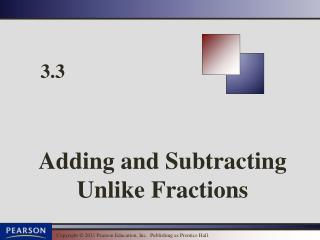DownloadDownload Presentation3.3

# 3.3

Download Presentation## 3.3

- - - - - - - - - - - - - - - - - - - - - - - - - - - E N D - - - - - - - - - - - - - - - - - - - - - - - - - - -
##### Presentation Transcript

1. Adding Unlike Fractions Adding or Subtracting Unlike Fractions Step 1: Find the LCM of the denominators of the fractions. This number is the least common denominator (LCD). Step 2: Write each fraction as an equivalent fraction whose denominator is the LCD. Step 3: Add or subtract the like fractions. Step 4: Write the sum or difference in simplest form.

2. Adding Unlike Fractions Multiples of 2: 2, 4, 6, 8, 10, … Multiples of 3: 3, 6, 9, 12, 15, … is in its simplest form.

3. Example Add: Step 1: The LCD of the denominators is 15. Step 2: Step 3: Step 4: The answer is in simplest form.

4. Example Add: The LCD of the denominators is 24.

5. Adding Unlike Fractions Practice Problem 1 Prime factorization for 6: 2, 3 Prime factorization for 18: 2, 3, 3 2, 3 3 P 192

6. Adding Unlike Fractions Practice Problem 2 Prime factorization for 6: 2, 3 Prime factorization for 9: 3, 3 2, 3 3 P 192

7. Adding Unlike Fractions Practice Problem 3 Prime factorization for 5: 5 Prime factorization for 9: 3, 3 5 3, 3 P 192

8. Adding Unlike Fractions Practice Problem 4 Prime factorization for 4: 2, 2 Prime factorization for 5: 5 Prime factorization for 10: 2, 5 2, 2 5 P 192

9. Subtracting Unlike Fractions EXAMPLE Subtract and simplify: SOLUTION Step 1: The LCD of 4 and 7 is 28 Step 2: Step 3: Step 4: is in its simplest form. P 193

10. Example Subtract: The LCD of the denominators is 33.

11. Example A freight truck has 1/4 ton of computers, 1/3 ton of televisions, and 3/8 ton of small appliances. Find the total weight of its load. To find the total weight, add the weights of the individual items.

12. Subtracting Unlike Fractions Prime factorization for 12: 2, 2, 3 Prime factorization for 24: 2, 2, 2, 3 P 193

13. Subtracting Unlike Fractions Practice Problem 6 Prime factorization for 10: 2, 5 Prime factorization for 7: 7 P 193

14. Subtracting Unlike Fractions Practice Problem 7 Prime factorization for 8: 2, 2, 2 Prime factorization for 6: 2, 3 2, 2, 2 3 P 194

15. Solving Problems by Adding or Subtracting Unlike Fractions EXAMPLE The slowest mammal is the three-toed sloth from South America. The sloth has an average ground speed of mph. In the trees, it can accelerate to mph. How much faster can a sloth travel in the trees?(Source: The Guiness Book of World Records) SOLUTION Step 1: The LCD of 10 and 100 is 100 Step 2: Step 3: Step 4: mph is in its simplest form. P 197

16. Solving Problems by Adding or Subtracting Unlike Fractions Practice Problem 8 To repair her sidewalk, a homeowner must pour small amounts of cement in three different locations. She needs of a cubic yard, of a cubic yard, and of a cubic yard for these locations. Find the total amount of cement the homeowner needs. SOLUTION Step 1: The LCD of 5, 10 and 15 is 30 Step 2: Step 3: Step 4: cubic yard is in its simplest form. P 194

17. DONE